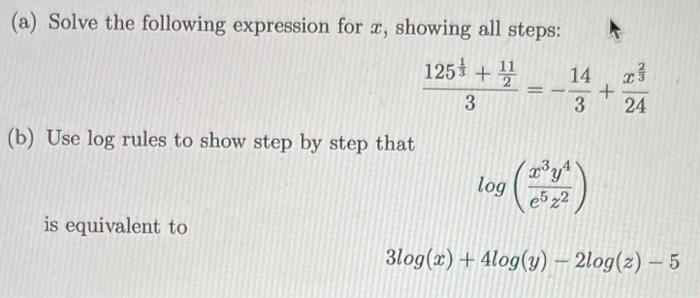Home / Expert Answers / Economics / a-solve-the-following-expression-for-x-showing-all-steps-frac-125-frac-1-3-frac-pa882

# (Solved): (a) Solve the following expression for $$x$$, showing all steps: $\frac{125^{\frac{1}{3}}+\frac ...(a) Solve the following expression for $$x$$, showing all steps: \[ \frac{125^{\frac{1}{3}}+\frac{11}{2}}{3}=-\frac{14}{3}+\frac{x^{\frac{2}{3}}}{24}$ (b) Use $$\log$$ rules to show step by step that $\log \left(\frac{x^{3} y^{4}}{e^{5} z^{2}}\right)$ is equivalent to $3 \log (x)+4 \log (y)-2 \log (z)-5$

We have an Answer from Expert